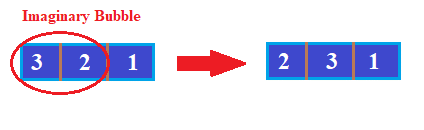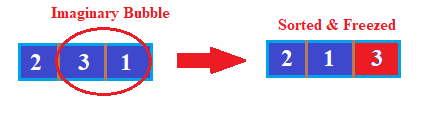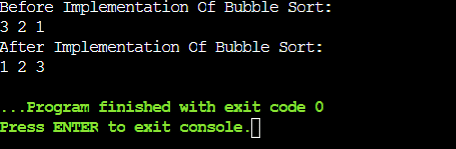# What is Bubble Sort In Java? Algorithms and Codes Explained

Do you know what is “Bubble Sort in Java programming language“? Have you ever thought about the working procedure of the Bubble Sort Algorithm in Java? Let us try to find out details of the algorithm by discussing the Bubble Sort example. Still, if you have any questions related to merge sort or any issues while solving your Java assignment then you can get  Java code helper by hiring the proficient experts at CodingZap.

But before we move ahead with the implementation of Bubble Sort code in Java programming language, we need to understand its basic concept. From where the Bubble Sort Algorithm concept comes from & why it is necessary to get a good knowledge of this topic, we will first discuss all of these.

## What Is Bubble Sort In Java Programming Language? Read Below

The basic concept Bubble Sort algorithm will be discussed first. We will unfold the answer to this question in a step-by-step format. First, we will focus on the term ‘Sorting Algorithm’. Clearing the concept of this term will help us a lot.

We all might know about sorting algorithms. Common sense might tell you that, it will be an algorithm that helps to do anything in a sorted manner. In this case, the sorting algorithm will only be worked on the integer data types or elements.

The sorting algorithms belong to the Algorithm section in computer science. But it not only belongs to the Algorithm section, but it also has some alignment with the Data Structure concept. Because one or more Data Structure elements are used in this topic.

For solving different problems, there are different types of sorting algorithms are present. Like, there are Merge Sort Algorithms, Quick Sort Algorithm, Heap Sort Algorithm & many others. Developers use their sense of algorithm while picking up one specific sorting algorithm for question-solving purposes.

If we are going to dive more deeply into the sorting algorithm section, then one article might not be enough to provide ample knowledge on this concept. We can say that the Bubble Sort algorithm is one of the algorithms from this group of sorting algorithms.

### Why is the need to use the word ‘Bubble’ there?

The bubble sort algorithm focuses on the sorting of the element. Many of the developers used the Bubble Sort algorithm for making a series of elements in an ascending or descending manner. But, the Bubble Sort algorithm works on ascending orders mainly.

In the Bubble Sort algorithm, there is no need to divide the array into two or more parts. Also, there is no need to find out the pivot element from the array. Two adjacent elements will create one imaginary bubble & in between them, the swapping process will be done.

In the Bubble Sort algorithm, the imaginary bubble creates where two adjacent elements are present. From that concept, the name was made. But one thing you should note is that no Divide & Conquer technique is used inside of this sorting algorithm.

In simple words, it working principle will be checking adjacent elements & based upon some condition swapping them. In this way, the largest number in the series will be on the right-hand side of the series. And the smallest element will be present on the left-hand side.

In an ascending manner, this workflow is being followed. And for descending manner, the opposite manner will be executed. Hence, the descending order Bubble Sort algorithm is sometimes called a Reverse Bubble Sort Algorithm.

## What Are The Steps Involved In Bubble Sort Java Algorithm? Get To Know

Now, we are sure that the previous discussion is not enough to clear the concept of the Bubble Sort algorithm in Java programming language. Now, we are going to discuss a detailed step-by-step process of the Bubble Sort algorithm in Java programming language.

To demonstrate the implementation of the Bubble Sort algorithm in Java programming language, we need to use a demo dataset. For that purpose, we have taken one set of arrays. In that array, there are three different elements present & all of them are in an unsorted manner.

In our demo array, there are three elements & they are 3, 2, 1. We should use the Bubble Sort algorithm to make a sorted array in a descending manner. Let us focus on the following steps for that purpose.

• ##### First Step:

In the array, the first two elements will come under consideration. The theory of the Bubble Sort algorithm tells that the adjacent two elements will play a crucial role. Here, the first two elements are 3 & 2. We will make an imaginary bubble around them.

Now, we should use one condition for checking purposes. If the second element is lesser than the first element, the swapping of the elements will happen. That means in this case, the second element is lesser than the first element. So, the swapping of the elements will happen.

Now, the element 3 will become the second element & the element 2 will become the first element in the array.• ##### Second Step:

After checking the first & second elements, we will focus on the second & third elements. In the array, the second element is Element 3 now & the third element is Element 1. We have to do again checking based upon the condition.

And we have found that the swapping is possible. And the imaginary bubble has again been created around these two elements. Now, the swapping will be done & element 1 will become the second element of the array.

Now, you might notice that element 3 will become the third & last element of the array. And it is the largest element in the array. So, the largest element is kept on the right-hand side of the array. Hence, this element will be frozen at that position.• ##### Third Step:

Now again the complete process will start from the beginning of the array. We will find out two different first & second elements inside the array. Now, the first element is Element 2 & the second element is Element 1. Now, checking will do in between these two elements.

Again, we should create one imaginary bubble around these two elements. And element 1 is less than the element 2. So, the swapping of these elements will happen in the array. Now, the element 1 will become the first element & the element 2 will become the second element.

Now, all the elements are in a sorted manner. Here, the sorting has been done using ascending order. If the series needs to be sorted in a descending manner, the condition to check the elements will be the opposite of what we are using now.We hope that the Bubble Sort algorithm in Java programming language is now quite clear. So, let us discuss one more important query before moving to the implementation process of the program in the Java programming language.

### Does Recursion Necessary In Bubble Sort In Java Programming Language?

One of the most important parts associated with every sorting algorithm is the use of recursion in the program. Because developers get confused with the use of the recursion inside of the different sorting algorithms.

Recursion is a method that helps to reduce the space complexity of any of the programs. The more space a program occupies, the more time it needs to complete. So, the very beginning steps of the time reduction are to do space reduction.

For that purpose, the recursion method is used where a certain function, calls the same function again & again inside of that function. But the use of the recursion is not universal. If anyone thinks to use the recursion method in every program, then he is committing one mistake.

The recursion method is not made for implementation in every program in computer science. We can take the Bubble Sort algorithm as an example. In the Bubble Sort algorithm, there is no use of the recursion method. The complete program is deployed using nested for loop.

But the Bubble Sort algorithm can be implemented using the recursion method. For that purpose, you should use the algorithm knowledge to modify the normal approach. But you should be aware of your programming requirement.

If any of the programs is not giving you the required time complexity with the Bubble Sort algorithm, you should switch to other sorting algorithms. Maybe the Bubble Sort algorithm will not apply to that problem. You have made some mistakes by choosing the Bubble Sort algorithm.

If the problem is not giving you the required time complexity with other sorting algorithms, then you try to implement a recursive Bubble Sort algorithm. So, the implementation of the Bubble Sort algorithm using the recursion theory is possible but limited to use.

##### What’s Next?

Now, we should move ahead with the implementation of Bubble Sort in Java programming language. We hope whatever we have discusses till now is clear to you. And you are ready to dive into the programming implementation of the Bubble Sort algorithm.

The implementation process of the Bubble Sort algorithm is very easy in the Java programming language. The bubble Sort algorithm in Java programming language is developed upon the concept of the function call & nested loop. We will start with the main function in the Java program.

The first step of the Bubble Sort algorithm in Java programming language is to declare the array of integers. Those integers’ values will be placed in an unsorted manner. In the program, we first printed the elements of the array before moving to the sorting.

After printing the elements, the function will be called for doing the Bubble Sort. Inside the function, nested for loops will be implemented. Inside the nested for loop, the condition to check the elements will be declared.

If the conditions are true, then the swapping of the elements will be done inside of the for loop. In this way, the complete process will be executed. For the swapping purpose, one local variable has also been declared inside of that function.

After all these operations are completed, we are good to go for the printing of the elements in the array. Now, the elements in the array are in a sorted manner. And the complete Bubble Sort algorithm in Java programming language is done.

##### Example:

```public class Main{ static void bubble(int[] a) {  // Implementation Of Bubble Sorting Algorithm

int x = a.length; // Getting The Size Of The Array

int t = 0;  // Local Variable Declaration

for(int i=0; i < x; i++){ // Outer For Loop Implementation

for(int j=1; j < (x-i); j++){  // Inner For Loop Implementation

if(a[j-1] > a[j]){  // Checking The Condition To Get Higher Value

t = a[j-1];  a[j-1] = a[j];  a[j] = t;}}}} // Swapping The Elements

public static void main(String[] args) {  int b[] ={3,2,1}; // Assigning The Array Value

System.out.println("Before Implementation Of Bubble Sort: "); // Printing Random Statement

for(int i=0; i < b.length; i++){  // For Loop For Printing The Previous Value

System.out.print(b[i] + " ");} // Printing Each & Every Elements

System.out.println();  // Next Line Implementation

bubble(b); // Calling The User-Defined Function From Main Function

System.out.println("After Implementation Of Bubble Sort: ");  // Printing Random Statement

for(int i=0; i < b.length; i++){  // For Loop For Printing The New Value

System.out.print(b[i] + " ");}}}  // Printing Each & Every Elements  ```

Let us try to find out the output of the above code. It will help to understand the working process of Bubble Sort in Java programming language.

##### Output:## Conclusion:

As we saw it is very important to know Bubble Sort in Java programming language.

In the bubble sort algorithm, the array concept is highly used. The array concept falls under the Data Structure concept. So, the Data Structure concept needs to be very clear before moving to the Bubble Sort algorithm in Java programming language.

Also, it is advisable to clear the basic Java programming language concept before jumping to this topic. Some of the important sections like calling a function, and sharing an array as the argument are necessary to know to perfectly implement the Bubble Sort algorithm in Java programming language.

So, hope you have liked this piece of article. Share your thoughts in the comments section and let us know if we can improve more.

Also, if you guys are looking to gain more knowledge about the concept of Java then you check out our below articles: